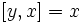# Lie subring whose sum with any subring is a subring not implies ideal

This article gives the statement and possibly, proof, of a non-implication relation between two Lie subring properties. That is, it states that every Lie subring satisfying the first Lie subring property (i.e., Lie subring whose sum with any subring is a subring) need not satisfy the second Lie subring property (i.e., ideal of a Lie ring)
View a complete list of Lie subring property non-implications | View a complete list of Lie subring property implications
Get more facts about Lie subring whose sum with any subring is a subring|Get more facts about ideal of a Lie ring

## Statement

There can exist a Lie ring$L$ and a subring$S$, such that the sum of$S$ and any subring of$L$ is a subring, but$S$ is not an ideal.

## Facts used

1. Subring of a Lie ring that is maximal as a subgroup

## Proof

Let$L$ be the Klein four-group with$x,y$ two of the non-identity elements. The four elements of$L$ are$0,x,y,x+y$. We define the Lie bracket as follows:$[x,y] = x$

This completely determines the Lie bracket:$[x,x+y] = [y,x] = [x+y,x] = [y,x+y] = [x+y,y] = x$.

Let$S$ be the subring of$L$ defined as$\{ 0,y \}$. The sum of$S$ with any subring of$L$ that does not contain$S$ is the whole ring$L$ (since$S$ is maximal as a subgroup of$L$). Also, the sum of$S$ with any subring of$L$ contained in$S$ is$S$. Thus, in any case, the sum of$S$ with any subring is a subring. (for more, see fact (1)).

However,$S$ is not an ideal of$L$, because$[y,x] = x$, and$x$ is not in$S$.